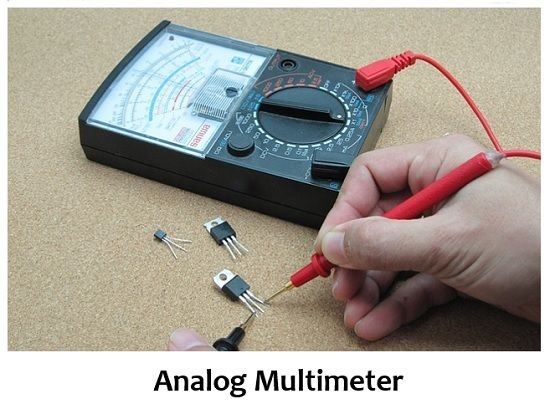# Difference Between Analog and Digital Multimeter

We know that multimeters are basically electronic test equipment, utilized for the purpose of determining various quantities like voltage, current, and resistance, etc. Multimeters are generally classified into two types, analog multimeter, and digital multimeter. The crucial difference between analog and digital multimeter lies in their way of representing the quantity being measured.

An analog multimeter displays the measuring quantity in an analog manner i.e., by a pointer deflection on a scale. As against, a digital multimeter displays the quantity in digital format i.e., by using digits. We will see some more parameters of differentiation between these two in this content.

## Content: Analog Multimeter Vs Digital Multimeter

### Comparison Chart

ParameterAnalog MultimeterDigital Multimeter
RepresentationIts display shows the measured quantity by deflection of pointer on scale.The display of digital multimeter represents the value in the form of digits.
Accuracy of measurementLowComparitively high
CostLowHigh
Physical sizeLargeComparitively small
UsabilityDifficultQuite easy
CalibrationManualAutomatic
Input resistanceVariable i.e., changes with range.Constant for all ranges.
Maximum permissible frequency rangeLowComparitively high
Susceptibility to electric noiseLowHigh

### Definition of Analog Multimeter

A type of multimeter that uses a needle on a scale to measure electrical quantities like voltage, current, and resistance is known as an analog multimeter. Basically whenever a quantity is measured by an analog multimeter then it displays the result in analog form, using a pointer that shows a specific value of the scale. The reading on the scale reflects the quantity to be measured.It is formed by a moving coil meter with a needle that shows the respective value on the scale.

Its basic principle of operation is such that the pointer shows deflection according to the force on it. Basically, it has a drum present between a pair of permanent magnets, and around the drum, a coil is wounded.

When a certain amount of current which is to be measured is passed through the coil, then this induces a magnetic field. Also, a magnetic field due to the permanent magnet exists that reacts with an induced magnetic field. Due to this, a resultant force results in deflection in the pointer attached to the drum.

The movement of this pointer is controlled by springs that apply, opposite force to its movement, in order to show balanced deflection. This spring is connected to the drum. In this way, the position of the needle on the scale represents the value of the current.

### Definition of Digital Multimeter

A digital multimeter is another type of multimeter that uses a digital display to represent the measured quantity in the form of digits.After the invention of digital multimeters, the use of analog multimeters turned somewhat limited since then. This is basically due to the advantageous factors associated with digital multimeters. The major component of a digital multimeter is the display unit along with encoders, analog to digital converters, signal conditioning circuits, etc.

Suppose the resistance offered by any particular resistor is to be measured. Then for this, current from a constant current source is allowed to flow through that resistor. The voltage existing across that resistor is amplified and provided to the ADC. Furthermore, the output of the ADC is then displayed by the LCD which represents the value of the unknown resistance.

## Key Differences Between Analog and Digital Multimeter

1. Analog multimeters display the result in analog form thus it does not require an analog to digital converter. However, a digital converter specifically needs an analog to digital converter inside it.
2. The accuracy of analog multimeters is comparatively low in comparison to the digital multimeter. As the digital multimeters generate more accurate results than analog ones.
3. Analog multimeters are used for the measurement of quantities like voltage, current, and resistance. While a digital multimeter along with these three measures impedance, capacitance, etc. and sometimes used for testing purposes.
4. Analog multimeters are calibrated manually, however, automatic calibration is the advantage of a digital multimeter.
5. Analog multimeters are quite difficult to use. Whereas digital multimeters provide ease of measurement.
6. The presence of components like encoder, ADC, LCD, logic circuits, etc. makes the circuit of a digital multimeter more complex than analog multimeters as these components are not present in it.
7. The physical size of an analog multimeter is larger than that of a digital multimeter.
8. Analog multimeter shows less susceptibility towards electric noise during measurement. While digital multimeters are more susceptible to electric noise.
9. The input impedance of analog meters is variable thus changes with range. Whereas it is constant in digital multimeter for all the ranges.
10. The permissible maximal measuring frequency is low for analog multimeters while it is quite high for digital meters.
11. To represent reverse polarity, an analog meter, the pointer deflects towards the left. While in digital multimeter, the reverse polarity is indicated by a negative sign.

### Conclusion

So, from this discussion, we can conclude that a multimeter alone performs the operation of ammeter, voltmeter, and ohmmeter as it can individually provide the values of all the quantities that are separately given by all these three instruments.